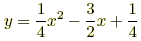index: click on a letter A B C D E F G H I J K L M N O P Q R S T U V W X Y Z A to Z index index: subject areas numbers & symbols sets, logic, proofs geometry algebra trigonometry advanced algebra & pre-calculus calculus advanced topics probability & statistics real world applications multimedia entrieswww.mathwords.com about mathwords website feedback

Axis of Symmetry of a Parabola

The line passing through the focus and vertex of a parabola. The axis of symmetry is perpendicular to the directrix.

 Example:This is a graph of the parabolawith all its major features labeled: axis of symmetry, focus, vertex, and directrix.